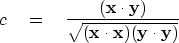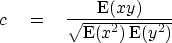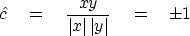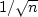Next: Coherency Up: CROSSCORRELATION AND COHERENCY Previous: CROSSCORRELATION AND COHERENCY

## Correlation

Correlation" is a concept similar to cosine. A cosine measures the angle between two vectors. It is given by the dot product of the two vectors divided by their magnitudes:(48)
This is the sample normalized correlation we first encountered on pageas a quality measure of fitting one image to another.

Formally, the normalized correlation is defined using x and y as zero-mean, scalar, random variables instead of sample vectors. The summation is thus an expectation instead of a dot product:(49)

A practical difficulty arises when the ensemble averaging is simulated over a sample. The problem occurs with small samples and is most dramatically illustrated when we deal with a sample of only one element. Then the sample correlation is(50)
regardless of what value the random number x or the random number y should take. For any n, the sample correlationscatters away from zero. Such scatter is called bias." The topic of bias and variance of coherency estimates is a complicated one, but a rule of thumb seems to be to expect bias and variance ofof aboutfor samples of size n. Bias, no doubt, accounts for many false discoveries,'' since cause-and-effect is often inferred from correlation.Next: Coherency Up: CROSSCORRELATION AND COHERENCY Previous: CROSSCORRELATION AND COHERENCY
Stanford Exploration Project
10/21/1998